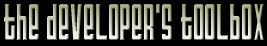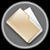This section of the archives stores flipcode's complete Developer Toolbox collection, featuring a variety of mini-articles and source code contributions from our readers.Gaussian Number Class   Submitted byThis contains my Gaussian number class. A gaussian number is a way to specify numeric quantities that are 'fuzzy' in nature. Gaussian numbers are expressed as a mean and standard deviation. In addition to this I also allow a minimum and maximum value for the generated number. The gaussian number class uses a deterministic random number generator to feed it, therefore all gaussian numbers which get generated are deterministic as well. This is a very powerful concept, deterministic fuzzy numbers! The gaussian number class has methods to set it via an ascii string, similar to atoi, or atof, in standard C notation. You can also retrieve a string representation of a gaussian number.

Gaussian number distribution conforms to the standard bell curve you see in statistics class. That means that if you specify a gausian number of 10 with a standard deviation of 2, you can, and will, get numbers much greater than 12 and much less than 8. In fact, you can get numbers which approach infinity on either side. It's just much less likely that you will get numbers outside of the range 8 to 12. Since, for the purposes of your simulation, you might require your input have some minimum and maximum limit, my gaussian number representation supports this.

Gaussian numbers have many uses in games and simulations. They are the absolute optimal numeric representation for any particle simulation, or input where you want to specify a fuzzy number instead of an exact one.

The syntax for a gaussian number is in four parts. Only the first part is required, the last 3 are optional. The four parts are:

Mean : The center of the bell curve in the gaussian distribution. Standard deviation : The average deviation between the mean value.

Min : The minimum value we will allow.
Max : The maximum value we will allow.

Some example gaussian numbers: 10:2 :A mean of 10 with a standard deviation of 2.
8 : A mean of 8 with a standard deviation of 0
7:3<4:10> : A mean of 7 with a standard deviation of 3 with a minimum value of 4 and a maximum value of 10. I've included the gaussian number class, the random number class, and a small test program to demonstrate the usage. Since the gaussian number is represented as a class, you can use them with templates to build ever more powerful data structures based on fuzzy number representations.

Currently browsing [gauss.zip] (5,747 bytes) - [gauss/gauss.cpp] - (3,942 bytes)

 ```#include #include #include #include #include #include "gauss.h"Gauss::Gauss(int seed) { mSecond = false; mMean = 1; mStandardDeviation = 0; mMin = -1e20f; mMax = 1e20f; Rand::Set(seed); // init random number generator };Gauss::Gauss(const char *str,int seed) { mSecond = false; mMean = 1; mStandardDeviation = 0; mMin = -1e20f; mMax = 1e20f; Rand::Set(seed); // init random number generator Set(str); };Gauss::~Gauss(void) { };float Gauss::Get(void) { float v = RandGauss()*mStandardDeviation + mMean; if ( v < mMin ) v = mMin; if ( v > mMax ) v = mMax; mCurrent = v; return v; };float Gauss::GetCurrent(void) const { return mCurrent; };void Gauss::Set(float m,float s) { mMean = m; mStandardDeviation = s; };// set directly. void Gauss::Set(float m,float s,float min,float max) { mMean = m; mStandardDeviation = s; mMin = min; mMax = max; }// Set from ascii string. const char * Gauss::Set(const char *arg) { char *end; strtogmd( (char *)arg, &end, mMean, mStandardDeviation, mMin, mMax ); return end; };float Gauss::GetMean(void) const { return mMean; };float Gauss::GetStandardDeviation(void) const { return mStandardDeviation; };void Gauss::SetMean(float m) { mMean = m; };void Gauss::SetStandardDeviation(float s) { mStandardDeviation = s; };// convert gaussian into valid gaussian string. void Gauss::GetString(char *scratch) const { if ( mStandardDeviation != 0 || mMin > -1e20f || mMax < 1e20f ) { if ( mMin > -1e20f || mMax < 1e20f ) sprintf(scratch,"%0.3f:%0.3f<%0.3f:%0.3f>",mMean,mStandardDeviation,mMin,mMax); else sprintf(scratch,"%0.3f:%0.3f",mMean,mStandardDeviation); } else { sprintf(scratch,"%0.3f",mMean); } };// generate fresh gaussian pair or return last valid one. float Gauss::RandGauss( void ) { if( mSecond ) { mSecond = false; return mGauss2; } float x1; float x2; float w; do { x1 = 2.0f * GetFloat() - 1.0f; x2 = 2.0f * GetFloat() - 1.0f; w = x1 * x1 + x2 * x2; } while ( w >= 1.0f ); w = sqrtf( (-2.0f * logf( w ) ) / w ); mGauss1 = x1 * w; mGauss2 = x2 * w; mSecond = true; return mGauss1; }; // convert string to gaussian number. Return code // indicates number of arguments found. int Gauss::strtogmd(const char* spec, char** end, float& mean, float& deviation, float& min, float& max ) const { char* pos; min = -1e20f; max = 1e20f; mean = (float) strtod( (char*) spec, &pos ); deviation = 0; if( pos == spec ) { mean = 0; if( end != 0 ) { *end = (char *)spec; } return 0; } if( *pos != ':' ) { if( end != 0 ) { *end = pos; } return 1; } char* devp = pos + 1; deviation = (float) strtod( devp, &pos ); if( pos == devp ) { if( end != 0 ) { *end = devp - 1; } return 1; } if( *pos != '[' && *pos != '<' ) { if( end != 0 ) { *end = pos; } return 2; } char* minp = pos + 1; char* maxp; min = (float) strtod( minp, &maxp ); if( *maxp != ',' && *maxp != ':' ) { if( end != 0 ) { *end = pos; } return 2; } ++maxp; char* endp; max = (float) strtod( maxp, &endp ); if( *endp != ']' && *endp != '>' ) { if( end != 0 ) { *end = pos; } return 2; } if( minp == maxp - 1 ) { min = -1e20f; } if( maxp == endp ) { max = 1e20f; } if( end != 0 ) { *end = endp + 1; } return 4; }; ```

Currently browsing [gauss.zip] (5,747 bytes) - [gauss/gauss.h] - (2,752 bytes)

 ```#ifndef GAUSS_H#define GAUSS_H// ** This class is a Gaussian Random Number Generator // ** A Gaussian number is expressed as having a mean and standard // ** deviation and generates random numbers which conform to the standard // ** bell curve distribution. Additionally this class applies an optional // ** minimum and maximum clamping range for all numbers generated. // ** The Guassian class inherits the deterministic random number class. // ** Therefore all gaussian sequences can be deterministic given the same // ** initial random number seed. // ** // ** The ASCII format for a gaussian number is as follows: // ** // ** mean:std // ** // ** Examples of valid gaussian numbers. // ** // ** 30 (Means 30 with a standard deviation of 0) // ** 30:5 (Means 30 with a standard deviation of +/- 5) // ** 30:5<10> (Means 30, stdev +/-5 minimum value of 10 // ** 30:5<10:40> (Means 30, stdev +/-5, min value 10 max value 40) #include "rand.h"// Implementation of gaussian numbers. class Gauss : public Rand { public: Gauss(int seed = 0); Gauss(const char *str,int seed=0); ~Gauss(void); float Get(void); // generate *deterministic* gaussian number. float GetCurrent(void) const; // last generated value. float GetMean(void) const; // return mean. float GetStandardDeviation(void) const; // return standard deviation. void GetString(char *str) const; // get string representation void Set(float m,float s); // set mean and standard deviation. void Set(float m,float s,float min,float max); // set mean, standard deviation, min and max values. const char * Set(const char *arg); // set from asciiz string, returns pointer past gauss number. void SetMean(float m); // set just the mean. void SetStandardDeviation(float s); // set just the standard deviation. float RandGauss( void ); // construct and return gaussian number. // convert string to gaussian number. Return code // indicates number of arguments found. int strtogmd(const char* spec, char** end, float& mean, float& deviation, float& min, float& max ) const; float GetMin(void) const { return mMin; }; float GetMax(void) const { return mMax; };private: float mMean; // gaussian number has mean and float mStandardDeviation; // standard deviation, also float mMin; // min/max clamping values float mMax; float mCurrent; // last got value. float mGauss1; // 1st gaussian float mGauss2; // 2nd gaussian bool mSecond; // have a computed second available. };#endif ```

Currently browsing [gauss.zip] (5,747 bytes) - [gauss/main.cpp] - (451 bytes)

 ```#include #include #include #include "gauss.h"void main(int argc,char **argv) { // Construct a deterministic Gaussian random number generator which has a mean value of 10, a standard deviation of 1, a clamped minimum value of // 8 and a clamped maximum value of 12 Gauss g("10:1<8:12>",0); for (int i=0; i<20; i++) { float v = g.Get(); printf("Sample: %d = %0.2f\n",i,v); }} ```

Currently browsing [gauss.zip] (5,747 bytes) - [gauss/rand.h] - (3,273 bytes)

 ```#ifndef RAND_H#define RAND_H//** Random number class and Random number pool class. This acts as a //** replacement for the stdlib rand() function. Why would you want to //** replace the rand() function? Because you often want a deteriminstic //** random number generator that is exactly the same regardless of what //** machine or compiler you build your code on. The stdlib rand() function //** is not guaraenteed to produce the same results on different stdlib //** implementations. //** //** You can also maintain any number of unique random number generators //** as state machines by instancing this rand class over and over again. //** //** The random number pool is a data structure which you allocate if you //** want to pull items from a data set, randomly, but without any //** duplications. A simple example would be a deck of cards. You would //** instantiate the random number pool as follows: //** //** RandPool deck(52); //** //** You would then pull cards from the deck as follows: //** //** bool shuffled; //** int card = deck.Get(shuffled); //** //** This will return a number between 0-51 (representing a card in the deck) //** without ever reporting the same card twice until the deck has been //** exhausted. If the boolean 'shuffled' is true, then the deck was //** re-shuffled on that call. This data structure has lots of uses in //** computer games where you want to randomly select data from a fixed //** pool size. //** //** This code submitted to FlipCode.com on July 23, 2000 by John W. Ratcliff //** It is released into the public domain on the same date. class Rand { public: Rand(int seed=0) { mCurrent = seed; }; // random number between 0 - 32767 int Get(void) { return(((mCurrent = mCurrent * 214013L + 2531011L) >> 16) & 0x7fff); }; // random number between 0.0 and 1.0 float GetFloat(void) { return float(Get())*(1.0f/32767.0f); }; void Set(int seed) { mCurrent = seed; };private: int mCurrent; };class RandPool { public: RandPool(int size,int seed) // size of random number bool. { mRand.Set(seed); // init random number generator. mData = new int[size]; // allocate memory for random number bool. mSize = size; mTop = mSize; for (int i=0; i

The zip file viewer built into the Developer Toolbox made use of the zlib library, as well as the zlibdll source additions.